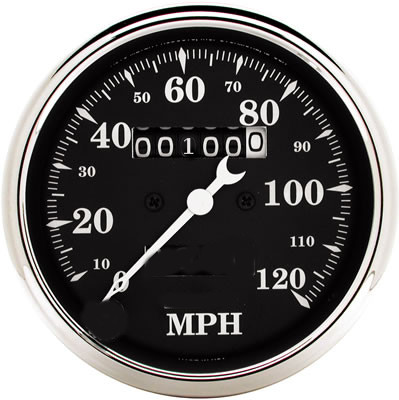# Automotive MathCalculus is taught all wrong and too late. The basics could be taught in the car on the way to Kindergarten.

Here is how it goes. Any kid who’s been in a car knows that the speedometer tells you how fast you are going, and the numbers in the odometer (mileage) tells you how far you’ve gone. Another way to say that is that the speedometer shows how quickly the odometer is changing. And another way to say that is that the speedometer is the “differential” of the odometer. To be fancy, we can use a small “∂” for differential and so we say:

$$Speedometer = ∂\,{Odometer}$$

That’s differential calculus. How things change. Subtraction.

Put another way again, the odometer tells you what the Sum total distance was after going all those speeds that the speedometer indicated. Instead of saying “Sum” with a big S we stretch it out into a long skinny S like this: $\int$ and we say

$$Odometer = \int { Speedometer }$$

That’s integral calculus. How changes accumulate. Addition.

Subtraction undoes addition. That is the Fundamental Theorem of Calculus. Duh. Next stop, Rocket Science !

THE END.